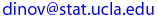## PIC 20 A,    Summer 2002

JAVA and Internet Programming, Computation & Visualization

# Homework Project  4

• [Project 4_1]   Create a class called Complex  for performing arithmetic on complex numbers.

Complex numbers have the form

realPart + imaginaryPart * i

where i is the imaginary unit [\sqrt(-1)]

• Use floating-point variables to represent the private data of the class. Provide constructor methods that enables an object of this class to be initialized when it is declared. Provide a no-argument constructor with default values in case no initializers are provided. Provide public methods for each of the following (as a drop-down list of options):

• Addition/Subtraction of two Complex numbers: (a+ib) +/- (c+id) = (a +/- c) + i(b +/- d)
• Multiplication of two Complex numbers: (a+ib) * (c+id) = (a*c - b*d) + i(ad + bc)
• Inversion (reciprocal) of a Complex number: (a+ib)-1 = 1 / (a + ib) = a/(a2 + b2) - i b/(a2 + b2)
• Division (divisor: a + ib != 0) of two Complex numbers: (c+id) / (a+ib) = (c+id) * (a+ib)-1
• Compute the magnitude of a complex number (this is a real number): | a + ib |2 = a2 + b2

Create an applet (or an application) that looks like this image and draws in the plane the entered complex numbers, as well as the results. You may use this as a starting point. If the result is a real number draw a thick line on the X-axis, starting at the origin. If the result is a complex number, graw it accordingly in color in the X-Y plane. [Proj4_1.java, Proj4_1.class,  (if applet) Proj4_1.html].

 Assignment 4, due date:   Wed., July 24, 2002 Last modified on by.

\Ivo D. Dinov, Ph.D., Departments of Statistics, Neurology, Program in Computing, UCLA School of Medicine/